# 小白学 Python 数据分析（19）：Matplotlib（四）常用图表（下）

2019/04/10 10:10## 散点图

matplotlib.pyplot.scatter(x, y, s=None, c=None, marker=None, cmap=None, norm=None, vmin=None, vmax=None, alpha=None, linewidths=None, verts=<deprecated parameter>, edgecolors=None, *, plotnonfinite=False, data=None, **kwargs)


• s ： 表示的是每个点的大小，如果只有一个数值的时候，则所有的点都是一样大的，也可以传入一个列表，这时候每个点的大小都不一样，散点图也就成了气泡图。
• c ： 表示点的颜色，如果只有一种颜色的时候，则每个点的颜色都会相同，也可以使用列表定义不同的颜色
• linewidths ： 表示每个散点的线宽
• edgecolors ： 每个散点外轮廓的颜色

import matplotlib.pyplot as plt
import numpy as np

# 处理中文乱码
plt.rcParams['font.sans-serif']=['SimHei']

x_data = np.array([2011,2012,2013,2014,2015,2016,2017])
y_data = np.array([58000,60200,63000,71000,84000,90500,107000])

plt.scatter(x_data, y_data, s = 100, c = 'green', marker='o', edgecolor='black', alpha=0.5, label = '产品销量')

plt.legend()

plt.savefig("scatter_demo.png")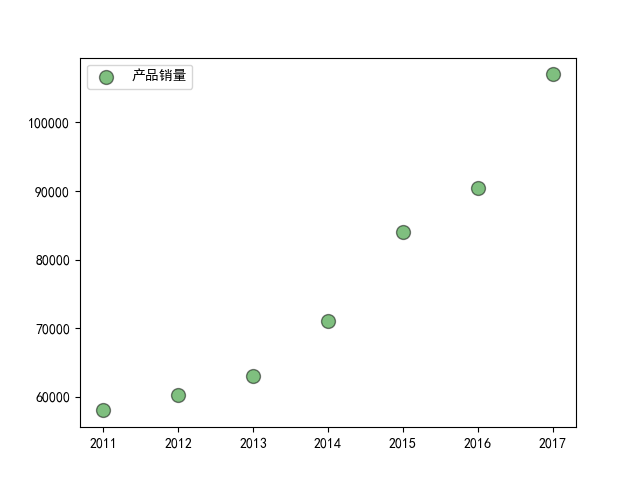## 气泡图

import matplotlib.pyplot as plt
import numpy as np

# 处理中文乱码
plt.rcParams['font.sans-serif']=['SimHei']

x_data = np.array([2011,2012,2013,2014,2015,2016,2017])
y_data = np.array([58000,60200,63000,71000,84000,90500,107000])

# 根据 y 值的不同生成不同的颜色
colors = y_data * 10
# 根据 y 值的不同生成不同的大小
area = y_data / 300

plt.scatter(x_data, y_data, s = area, c = colors, marker='o', edgecolor='black', alpha=0.5, label = '产品销量')

plt.legend()

plt.savefig("scatter_demo1.png")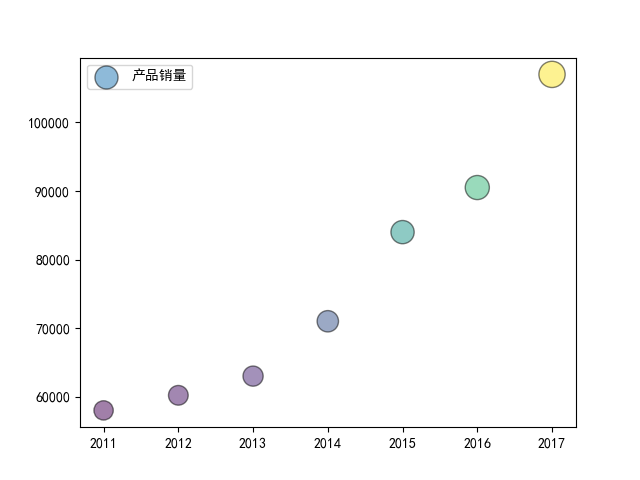## 堆叠图

plt.stackplot(x, y, labels, colors)


stackplot() 堆叠图的语法比较简单，这里就不多介绍了，看过前面的同学应该一眼就能明白，接下来我们看个示例：

import matplotlib.pyplot as plt

# 处理中文乱码
plt.rcParams['font.sans-serif']=['SimHei']

x_data = [2011,2012,2013,2014,2015,2016,2017]
y_data = [58000,60200,63000,71000,84000,90500,107000]
y_data_1 = [78000,80200,93000,101000,64000,70500,87000]

plt.title(label='xxx 公司 xxx 产品销量')

plt.stackplot(x_data, y_data, y_data_1, labels=['产品销量', '用户增长数'])

plt.legend()

plt.savefig("stackplot_demo.png")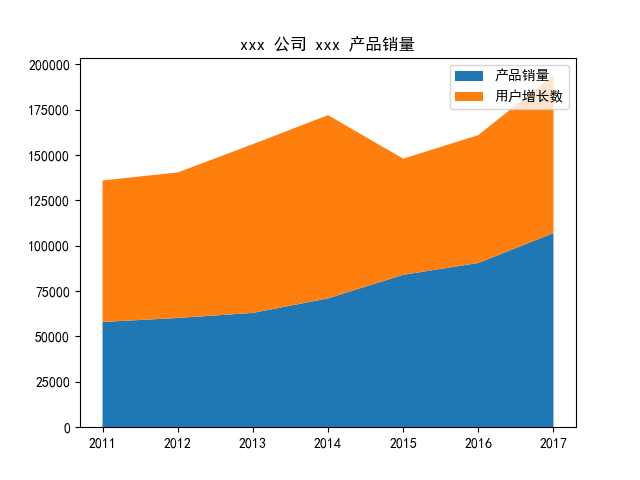## 雷达图

plt.polar(theta, r, **kwargs)

• theta ： 每一个点在极坐标系中的角度
• r ： 每一个点在极坐标系中的半径

import numpy as np
import matplotlib.pyplot as plt

# 中文和负号的正常显示
plt.rcParams['font.sans-serif']=['SimHei']
plt.rcParams['axes.unicode_minus'] = False

# 使用ggplot的绘图风格
plt.style.use('ggplot')

# 构造数据
values = [3.2, 2.1, 3.5, 2.8, 3]
feature = ['攻击力', '防御力', '恢复力', '法术强度', '生命值']

N = len(values)
# 设置雷达图的角度，用于平分切开一个圆面
angles = np.linspace(0, 2 * np.pi, N, endpoint=False)

# 为了使雷达图一圈封闭起来，需要下面的步骤
values = np.concatenate((values, [values]))
angles = np.concatenate((angles, [angles]))

# 绘图
fig = plt.figure()
# 这里一定要设置为极坐标格式
# 绘制折线图
ax.plot(angles, values, 'o-', linewidth=2)
# 填充颜色
ax.fill(angles, values, alpha=0.25)
# 添加每个特征的标签
ax.set_thetagrids(angles * 180 / np.pi, feature)
# 设置雷达图的范围
ax.set_ylim(0, 5)
# 添加标题
plt.title('游戏人物属性')
# 添加网格线
ax.grid(True)
# 显示图形
plt.savefig('polar_demo.png')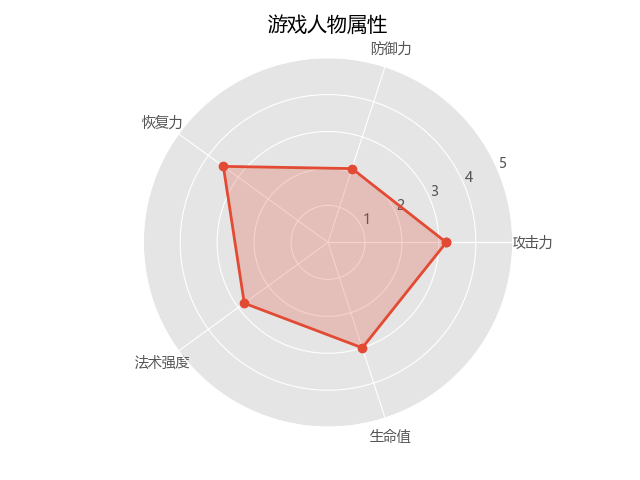## 饼图

matplotlib.pyplot.pie(x, explode=None, labels=None, colors=None, autopct=None, pctdistance=0.6, shadow=False, labeldistance=1.1, startangle=None, radius=None, counterclock=True, wedgeprops=None, textprops=None, center=(0, 0), frame=False, hold=None, data=None)


• x : array-like
• explode : array-like, optional, default: None(设置饼图偏离)
• labels : list, optional, default: None(标签)
• colors : array-like, optional, default: None(颜色设置)
• autopct : None (default), string, or function, optional(设置百分比显示)
• pctdistance : float, optional, default: 0.6(百分比距圆心的位置)
• shadow : bool, optional, default: False(设置阴影)
• labeldistance : float, optional, default: 1.1(标签距圆心的距离)
• startangle : float, optional, default: None(开始位置的旋转角度，第一个分类是在右45度位置)
• radius : float, optional, default: None(设置半径的大小)
• counterclock : bool, optional, default: True(是否逆时针)
• wedgeprops : dict, optional, default: None(内外边界设置)
• textprops : dict, optional, default: None(文本设置)
• center : list of float, optional, default: (0, 0)(设置中心位置)
• frame : bool, optional, default: False(是否显示饼图背后图框)

import matplotlib.pyplot as plt

# 中文和负号的正常显示
plt.rcParams['font.sans-serif']=['SimHei']
plt.rcParams['axes.unicode_minus'] = False

# 数据
edu = [0.2515,0.3724,0.3336,0.0368,0.0057]
labels = ['中专','大专','本科','硕士','其他']

# 让本科学历离圆心远一点
explode = [0,0,0.1,0,0]

# 将横、纵坐标轴标准化处理，保证饼图是一个正圆，否则为椭圆
plt.axes(aspect='equal')

# 自定义颜色
colors=['#9999ff','#ff9999','#7777aa','#2442aa','#dd5555'] # 自定义颜色

# 绘制饼图
plt.pie(x=edu,  # 绘图数据
explode = explode,  # 突出显示大专人群
labels = labels,  # 添加教育水平标签
colors = colors,  # 设置饼图的自定义填充色
autopct = '%.1f%%',  # 设置百分比的格式，这里保留一位小数
)

# 添加图标题
plt.title('xxx 公司员工教育水平分布')

# 保存图形
plt.savefig('pie_demo.png')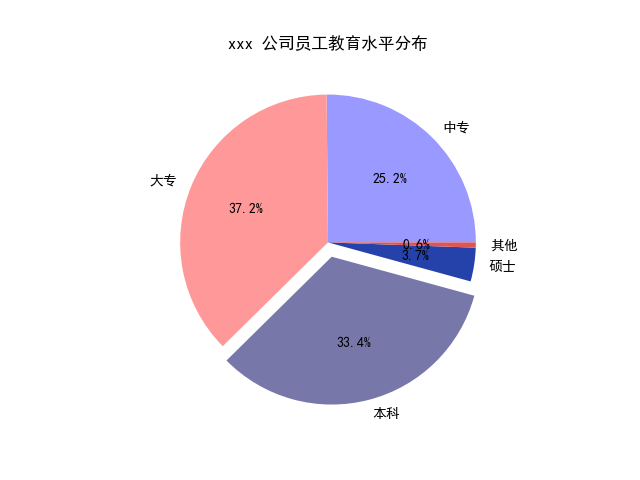## 环形图

import matplotlib.pyplot as plt

# 中文和负号的正常显示
plt.rcParams['font.sans-serif']=['SimHei']
plt.rcParams['axes.unicode_minus'] = False

# 数据
edu = [0.2515,0.3724,0.3336,0.0368,0.0057]
labels = ['中专','大专','本科','硕士','其他']

# 让本科学历离圆心远一点
explode = [0,0,0.1,0,0]

# 将横、纵坐标轴标准化处理，保证饼图是一个正圆，否则为椭圆
plt.axes(aspect='equal')

# 自定义颜色
colors=['#9999ff','#ff9999','#7777aa','#2442aa','#dd5555'] # 自定义颜色

# 绘制饼图
plt.pie(x=edu,  # 绘图数据
explode = explode,  # 突出显示大专人群
labels = labels,  # 添加教育水平标签
colors = colors,  # 设置饼图的自定义填充色
autopct = '%.1f%%',  # 设置百分比的格式，这里保留一位小数
wedgeprops = {'width': 0.3, 'edgecolor':'green'}
)

# 添加图标题
plt.title('xxx 公司员工教育水平分布')

# 保存图形
plt.savefig('pie_demo1.png')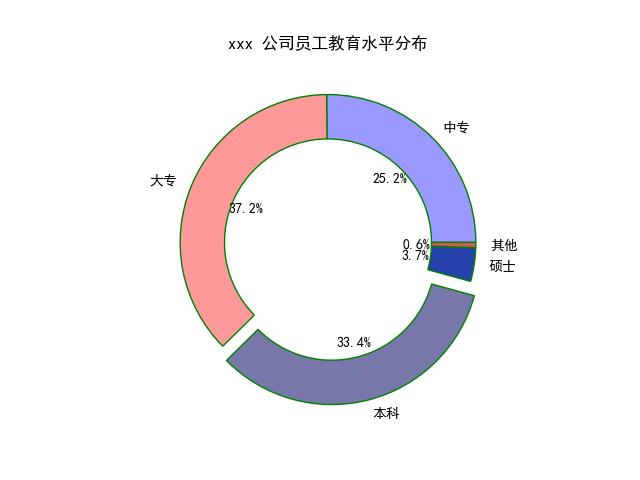## 热力图

plt.imshow(x, cmap)


import numpy as np
import matplotlib.pyplot as plt

x = np.random.rand(10, 10)
plt.imshow(x, cmap=plt.cm.hot)

# 显示右边颜色条
plt.colorbar()

plt.savefig('imshow_demo.png')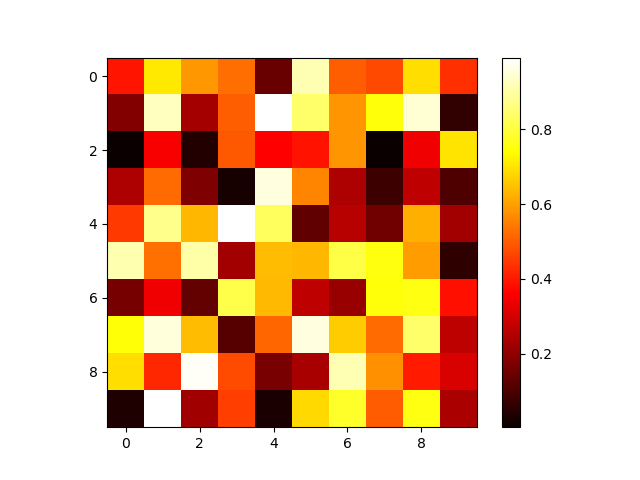Matplotlib 的常用图表到这里就要结束了，接下来，我们会接着介绍我们之前说过的 pyecharts 的常用图表。

## 参考

https://blog.csdn.net/kun1280437633/article/details/80841364

https://blog.csdn.net/claroja/article/details/72911155

0
0 收藏

### 作者的其它热门文章0 评论
0 收藏
0